American Journal of Theoretical and Applied Statistics
Volume 4, Issue 6, November 2015, Pages: 534-538

Solution of Linear and Nonlinear Schrodinger Equations by Combine Elzaki Transform and Homotopy Perturbation Method

Mohannad H. Eljaily1, Tarig M. Elzaki1, 2

1Department of Mathematic, Faculty of Sciences, Sudan University of Sciences and Technology, Khartoum, Sudan

2Mathematics Department, Faculty of Sciences and Arts-Alkamil, University of  Jeddah, Jeddah ,Saudi Arabia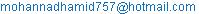(M. H. Eljaily)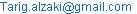(T. M. Elzaki)

Mohannad H. Eljaily, Tarig M. Elzaki. Solution of Linear and Nonlinear Schrodinger Equations by Combine Elzaki Transform and Homotopy Perturbation Method.American Journal of Theoretical and Applied Statistics.Vol.4, No. 6, 2015, pp. 534-538. doi: 10.11648/j.ajtas.20150406.24

Abstract: In this paper, the homotopy perturbation method (HPM) and ELzaki transform are employed to obtain the approximate analytical solution of the Linear and Nonlinear Schrodinger Equations. The proposed method is an elegant combination of the new integral transform "ELzaki Transform" and the homotopy perturbation method. This method finds the solution without any discretization, linearization or restrictive assumptions and avoids the round-off errors,the results reveal that the ETHPM is very efficient, simple and can be applied to other nonlinear problems.

Keywords: ELzaki Transform, Homotopy Perturbation Method, He’s Polynomials,
Linear and Nonlinear Schrodinger Equations

Contents

1. Introduction

The investigation of the exact solutions to nonlinear equations plays an important role in the study of nonlinear physical phenomena.The linear and nonlinear Schrodinger equations have been widely used in various application areas, e.g., quantum mechanics, optics, seismology and plasma physics. Since analytic approaches to the Schrodinger equation have limited applicability in science and engineering problems, there is a growing interest in exploring new methods to solve the equation more accurately and efficiently.In recent years, many research workers have paid attention to study the solutions of nonlinear partial differential equations by using various methods.Among thesethe Adomian decomposition method Hashim, Noorani, Ahmed.Bakar, Ismail and Zakaria, (2006), the tanh method, the homotopy perturbation method Sweilam, Khader (2009), Sharma and GirirajMethi (2011), Jafari, Aminataei (2010), (2011), the differential transform method (2008), homotopy perturbation transform method and the variational iteration method.He [12–25] developed the homotopy perturbation method (HPM) by merging the standard homotopy and perturbation for solving various physical problems. It is worth mentioning that the HPM is applied without any discretization, restrictive assumption or transformation and is free from round off errors. Homotopy perturbation transform method and the variational iteration method. Various ways have been proposed recently to deal with these nonlinearities; one of these combinations is ELzaki transform and homotopy perturbation method. ELzaki transform is a useful technique for solving linear differential equations, but this transform is totally incapable of handling nonlinear equations  because of the difficulties that are caused by the nonlinear terms. This paper uses homotopy perturbation method to decompose the nonlinear term, so that the solution can be obtained by iteration procedure. This means that we can use both ELzaki transform and homotopy perturbation methods to solve many nonlinear problems.The main aim of this paper is to consider the effectiveness of the Elzaki transform homotopy perturbation method in solving Linear and Nonlinear Schrodinger Equations. The HPM and He’s polynomials and is mainly due to Ghorbani [8,9].This method provides the solution in a rapid convergent series which may leads the solution in a closed form. The advantage of this method is its capability of combining two powerful methods for obtaining exact solutions for nonlinear equations. Inspired and motivated by the ongoing research in this area, we apply the ETHPM in solving the linear and nonlinear Schrodinger equations to show the simplicity and straightforwardness of the method.

2. Basic Idea

To illustrate the basic idea of this method, we consider a general nonlinear non homogeneous partial differential equation with initial conditions of the form: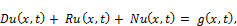(1)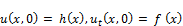Where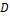is the second order linear differential operator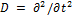, is the linear differential operator of less order than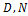represents the general non-linear differential operator and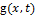is the source term.

Taking Elzaki transform(denoted throughout this paper by E) on both sides of Eq. (1), to get: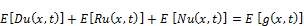(2)

Using the differentiation property ofElzakitransform and above initial conditions, we have: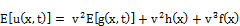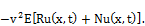(3)

Operating with the Elzaki inverse on both sides of Eq.(3) gives: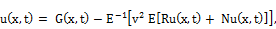(4)

Where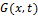represents the term arising from the source term and the prescribed initial condition.

Now, we apply the homotopy perturbation method;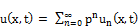(5)

And the nonlinear term can be decomposed as: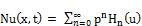(6)

Where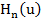are He’s polynomial and given by: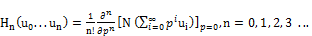(7)

Substituting Eqs. (6) and (5) in Eq. (4) we get: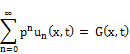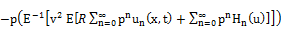(8)

Which is the coupling of the Elzaki transform and the homotopy perturbation method using He’s polynomials. Comparing the coefficient of like powers of p, the following approximations are obtained: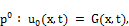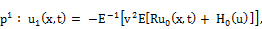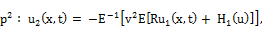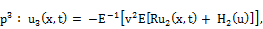Then the solution is;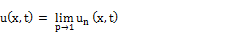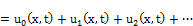(9)

3. Applications

In this section, the effectiveness and the usefulness of ELzaki transform homotopy perturbation method (ETHPM) are demonstrated by finding exact solutions of Linear and Nonlinear Schrodinger Equations.

Example 3.1. Consider the following linear homogeneous Schrodinger Equation;(10)

With the initial condition;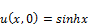(11)

Applying the Elzaki transform of both sides of Eq. (10),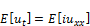(12)

Using the differential property of Elzaki transform Eq.(12) can be written as: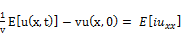(13)

Using initial condition (11), Eq. (13) can be written as: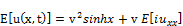(14)

The inverse Elzaki transform implies that: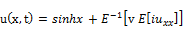(15)

Now, we apply the homotopy perturbation method, we get: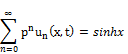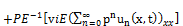(16)

Comparing the coefficient of like powers of p, the following approximations are obtained;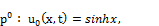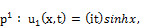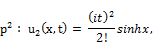Proceeding in a similar manner, we have: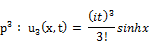Therefore the solution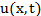is given by: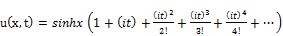(17)

In series form, and,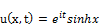(18)

In closed form.

Example 3.2. Consider the following nonlinear homogeneous Schrodinger Equation,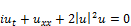(19)

With the initial condition;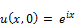(20)

Applying the Elzaki transform of both sides of Eq. (19),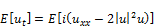(21)

Using the differential property of Elzaki transform Eq. (21) can be written as: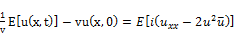(22)

Where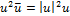and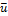is the conjugate of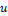.

Using initial condition (20), Eq. (22) can be written as: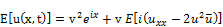(23)

The inverse Elzaki transform implies that: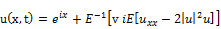(24)

Now, we apply the homotopy perturbation method, we get: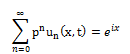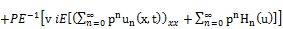(25)

Where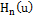are He’s polynomial [8, 9] that represents the nonlinear terms. The first few components of He’s polynomials, are given by: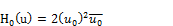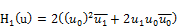Comparing the coefficients of like powers of p, we have: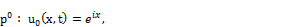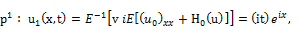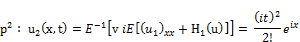Proceeding in a similar manner, we have: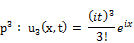Therefore the solutionis given by: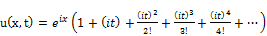(26)

In series form, and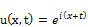(27)

In closed form.

Example 3.3. Consider the following nonlinear inhomogeneous Schrodinger Equation,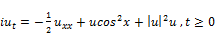(28)

With the initial condition;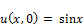(29)

Taking the Elzaki transform on both sides of Eq. (28) subject to the initial condition (29),

we have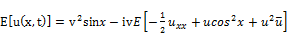(30)

Whereandis the conjugate of.

The inverse Elzaki transform implies that: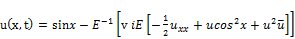(31)

Now, we apply the homotopy perturbation method, we get: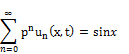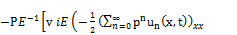(32)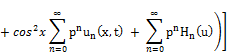Comparing the coefficient of like powers of p, we have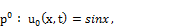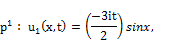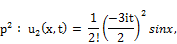Proceeding in a similar manner, we have: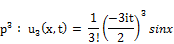Therefore the solutionis given by: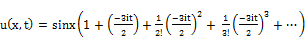(33)

In series form, and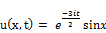(34)

In closed form.

4. Conclusions

In this paper, the ETHPM has been successfully applied to find the solution of the linear and nonlinear Schrodinger equations with initial conditions. The method is reliable and easy to use. The results show that the ETHPM is a powerful andefficient technique in finding exact and approximate solutions of nonlinear differential equations. By using this method we obtain a new, efficient recurrent relation, to solve linear and nonlinear Schrodinger equations, this means that ETHPM provide highly accurate numerical solutions for nonlinear problems in comparison with other method.

References

1. Tarig M. Elzaki (2011), The New Integral Transform "ELzaki Transform", Global Journal of Pure and Applied Mathematics, Vol.7, No.1,pp57-64.
2. Tarig M. Elzaki and Salih M. Elzaki (2011), Applications of New Transform "ELzaki Transform" to Partial Differential Equations, Global Journal of Pure and Applied Mathematics, Vol.7, No.1,pp65-70.
3. Tarig M. Elzaki and Eman M. A. Hilal (2012), Homotopy Perturbation and ELzaki Transform for solving Nonlinear Partial Differential equations, Mathematical Theory and Modeling, Vol.2,No.3, pp33-42.
4. J.H. He, Homotopy perturbation technique, Computer Methods in Applied Mechanics and Engineering, 178(1999):257-262.
5. N.H. Sweilam and M.M. Khader, Exact solutions of some coupled nonlinear partial differential equations using the homotopy perturbation method, Computers & Mathematics with Applications, 58(2009):2134 2141.
6. A.M. Wazwas, A study on linear and non-linear Schrodinger equations by the variational iteration method, Chaos,Solitions and Fractals, 37(4)(2008):1136-1142.
7. B. Jazbi and M. Moini, Application of He’s homotopy perturbation method for Schrodinger equation, Iranian Journalof Mathematical Sciences and Informatics, 3(2)(2008):13-19.
8. A. Ghorbani and J. Saberi-Nadjafi, He’s homotopy perturbation method for calculating adomian polynomials, Inter-national Journal of Nonlinear Sciences and Numerical Simulation, 8(2007):229-232.
9. A. Ghorbani, Beyondadomian’s polynomials: He polynomials, Chaos Solitons Fractals, 39(2009):1486-1492.
10. S.T. Mohyud-Din, M.A. Noor and K.I. Noor, Traveling wave solutions of seventh-order generalized KdV equation using He’s polynomials, International Journal of Nonlinear Sciences and Numerical Simulation, 10(2009):227-233.
11. Y. Khan and Q. Wu, Homotopy perturbation transform method for nonlinear equations using He’s polynomials, Computer and Mathematics with Applications, 61(8)(2011):1963-1967.
12. J.H. He, Homotopy perturbation method: a new nonlinear analytical technique, Applied Mathematics and Computa- tion, 135(2003):73-79.
13. J.H. He, Comparison of homotopy perturbation method and homotopy analysis method, Applied Mathematics and Computation, 156(2004):527539.
14. J.H. He, The homotopy perturbation method for nonlinear oscillators with discontinuities, Applied Mathematics and Computation, 151(2004):287292.
15. J.H. He, Homotopy perturbation method for bifurcation of nonlinear problems, International Journal of Nonlinear Sciences and Numerical Simulation, 6(2005):207-208.
16. J.H. He, Some asymptotic methods for strongly nonlinear equation, International Journal of Modern Physics, 20(2006):1144-1199.
17. J.H. He, Homotopy perturbation method for solving boundary value problems, Physics Letters A, 350(2006):87-88.
18. Rafei and D.D. Ganji, Explicit solutions of helmhotz equation and fifth order KdV equation using homotopy perturbation method, International Journal of Nonlinear Sciences and Numerical Simulation, 7(2006):321-328.
19. A.M. Siddiqui, R. Mahmood and Q.K. Ghori, Thin film flow of a third grade fluid on a moving belt by He’s homotopy perturbation method, International Journal of Nonlinear Sciences and Numerical Simulation, 7(2006):7-14.
20. D.D. Ganji, The applications of He’s homotopy perturbation method to nonlinear equation arising in heat transfer, Physics Letters A, 335(2006):337–341.
21. L. Xu, He’s homotopy perturbation method for a boundary layer equation in unbounded domain, Computers &Math- ematics with Applications, 54(2007):1067-1070.
22. J.H. He, An elementary introduction of recently developed asymptotic methods and nanomechanics in textile engi- neering, International Journal of Modern Physics, 22(2008):3487-3578.
23. J.H. He, Recent developments of the homotopy perturbation method, Topological Methods in Nonlinear Analysis, 31(2008):205-209.
24. E. Hesameddini and H. Latifizadeh, An optimal choice of initial solutions in the homotopy perturbation method, International Journal of Nonlinear Sciences and Numerical Simulation, 10(2009):1389-1398.
25. E. Hesameddini and H. Latifizadeh, A new vision of the He’s homotopy perturbation method, International Journal of Nonlinear Sciences and Numerical Simulation, 10(2009):1415-1424.
26. J. Biazar, M. GholamiPorshokuhi and B. Ghanbari, Extracting a general iterative method from an adomiandecom- position method and comparing it to the variational iteration method, Computers & Mathematics with Applications, 59(2010):622-628.
27. Tarig. M. Elzaki and J. Biazar, Homotopy Perturbation Method and Elzaki Transform for Solving System of Nonlinear Partial Differential Equations, World Applied Sciences Journal 24 (7): 944-948, 2013.
28. E. Hesameddini and N. Abdollahy, Homotopy perturbation and Elzaki transform for solving Sine-Gorden and Klein-Gorden equations, Iranian J. of Numerical Analysis and Optimization Vol 3, No. 2, (2013), pp 33-46.
29. Prem Kiran G. Bhadane, V. H. Pradhan, Elzaki Transform Homotopy Perturbation Method for Solving Fourth Order Parabolic PDE with Variable Coefficients, P. G. Bhadane et al Int. Journal of Engineering Research and Applications, Vol. 3, Issue 6, 2013, pp.2170-2173.

 Contents 1. 2. 3. 4.
Article ToolsAbstractPDF(179K)